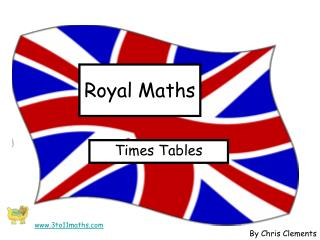DownloadDownload PresentationRoyal Maths

# Royal Maths

Télécharger la présentation## Royal Maths

- - - - - - - - - - - - - - - - - - - - - - - - - - - E N D - - - - - - - - - - - - - - - - - - - - - - - - - - -
##### Presentation Transcript

1. Royal Maths Times Tables www.3to11maths.com By Chris Clements

2. Louise Windsor 3 x 8 = 3 x 5 = 10 x 2 = 6 x 5 = 3 x 4 = Peter Phillips Zara Phillips Sarah Ferguson 10 x 10 = 2 x 8 = 3 x 5 = Princess Beatrice 8 x 5 = Countess Sophie 4 x 2 = 2 x 5 = The Queen Kate Middleton Prince Harry Prince Phillip Princess Anne Prince William Mark Phillips Autumn Philips Princess Diana Prince William Camilla Parker-Bowles Princess Eugenie James Windsor 9 x5 = Prince Andrew Timothy Laurence Prince Edward Prince Charles 1 x 9 = 7 x 4 = 0 x 6 = 3 x 10 = 9 x 2 = 10 x 5 = 7 x 10 = 5 x 5 = 10 x 8 = 8 x 4 = 0 x 10 = 8 15 24 50 18 100 30 10 12 28 80 67 16 67 0 9 30 40 20 25 Mike Tindall Savannah Phillips 70 32 0 45 9 21 3 x 3 = 3 x 7 = Sheet 1 www.3to11maths.com

3. Louise Windsor 9 x 3 = 9 x 5 = 6 x 5 = 7 x 8 = 7 x 6 = Peter Phillips Zara Phillips Sarah Ferguson 9 x 9 = 4 x 8 = 3 x 5 = Princess Beatrice 8 x 8 = Countess Sophie 6 x 8 = 6 x 6 = The Queen Kate Middleton Prince Harry Prince Phillip Princess Anne Prince William Mark Phillips Autumn Philips Princess Diana Prince William Camilla Parker-Bowles Princess Eugenie James Windsor 9 x 0 = Prince Andrew Timothy Laurence Prince Edward Prince Charles 7 x 9 = 8 x 0 = 3 x 8 = 9 x 7 = 7 x 4 = 5 x 8 = 9 x 4 = 7 x 7 = 8 x 9 = 6 x 3 = 4 x 7 = 48 45 27 40 28 81 56 36 42 0 72 67 32 67 24 63 63 64 30 49 Mike Tindall Savannah Phillips 36 18 28 0 48 0 8 x 6 = 0 x 10 = Sheet 2 www.3to11maths.com

4. Louise Windsor 9 x 7 = 7 x 6 = 5 x 32 = 11 x 12 = 12 x 4 = Peter Phillips Zara Phillips Sarah Ferguson 12 x 12 = 7 x 12 = 3 x 5 = Princess Beatrice 4 x 23 = Countess Sophie 8 x 8 = 6 x 6 = The Queen Kate Middleton Prince Harry Prince Phillip Princess Anne Prince William Mark Phillips Autumn Philips Princess Diana Prince William Camilla Parker-Bowles Princess Eugenie James Windsor 12 x 15 = Prince Andrew Timothy Laurence Prince Edward Prince Charles 3 x 80 = 11 x 11 = 50 x 6 = 30 x 30 = 7 x 7 = 7 x 8 = 57 x 0 = 10 x 12 = 4 x 7= 99 x 4 = 23 x 5 = 64 42 63 56 49 144 132 36 48 121 28 67 84 67 300 240 900 92 160 120 Mike Tindall Savannah Phillips 0 396 115 180 420 96 60 x 7 = 24 x 4 = Sheet 3 www.3to11maths.com